GeeksforGeeks App
Open AppBrowser
Continue

# Introduction to Vaex in Python

Working on Big Data has become very common today, So we require some libraries which can facilitate us to work on big data from our systems (i.e., desktops, laptops) with instantaneous execution of Code and low memory usage.

Vaex is a Python library which helps us achieve that and makes working with large datasets super easy. It is especially for lazy Out-of-Core DataFrames (similar to Pandas). It can visualize, explore, perform computations on big tabular datasets swiftly and with minimal memory usage.

## Installation:

Using Conda:

`conda install -c conda-forge vaex`

Using pip:

`pip install --upgrade vaex`

## Why Vaex?

Vaex helps us work with large datasets efficiently and swiftly by lazy computations, virtual columns, memory-mapping, zero memory copy policy, efficient data cleansing, etc. Vaex has efficient algorithms and it emphasizes aggregate data properties instead of looking at individual samples. It is able to overcome several shortcomings of other libraries (like:- pandas). So, Let’s Explore Vaex:-

For large tabular data, the reading performance of Vaex is much faster than pandas. Let’s analyze by importing same size dataset with both libraries. Link to the dataset

## Python3

 `import` `pandas as pd``%``time df_pandas ``=` `pd.read_csv(``"dataset1.csv"``)`

Output:

`Wall time: 1min 8s`

## Python3

 `import` `vaex``%``time df_vaex ``=` `vaex.``open``(``"dataset1.csv.hdf5"``)`

Output:

`Wall time: 1.34 s`

Vaex took very little time to read the same size dataset as compared to pandas:

## Python3

 `print``(``"Size ="``)``print``(df_pandas.shape)``print``(df_vaex.shape)`

Output:

```Size =
12852000, 36
12852000, 36```

## Vaex does computations lazily

Vaex uses a lazy computation technique (i.e., compute on the fly without wasting RAM). In this technique, Vaex does not do the complete calculations, instead, it creates a Vaex expression, and when printed out it shows some preview values. So Vaex performs calculations only when needed else it stores the expression. This makes the computation speed of Vaex exceptionally fast. Let’s Perform an example on a simple computation:

Pandas DataFrame:

## Python3

 `%``time df_pandas[``'column2'``] ``+` `df_pandas[``'column3'``]`

Output: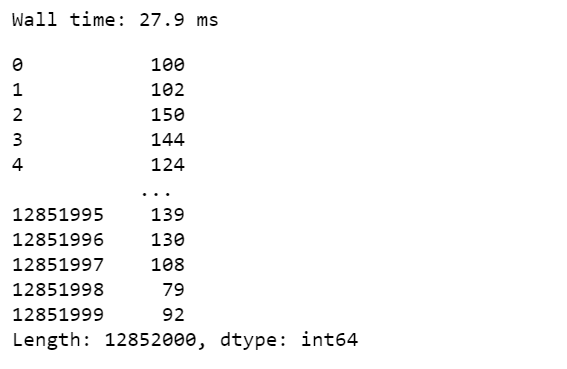Vaex DataFrame:

## Python3

 `%``time df_vaex.column2 ``+` `df_vaex.column3`

Output: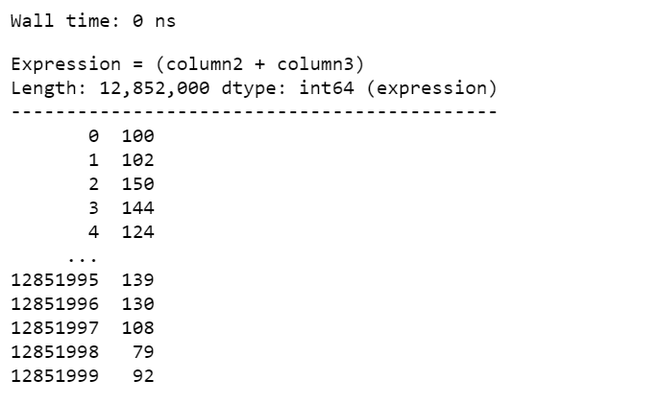## Statistics Performance:

Vaex can calculate statistics such as mean, sum, count, standard deviation, etc., on an N-dimensional grid up to a billion (109) objects/rows per second. So, Let’s Compare the performance of pandas and Vaex while computing statistics:-

Pandas Dataframe:

## Python3

 `%``time df_pandas[``"column3"``].mean()`

Output:

```Wall time: 741 ms
49.49811570183629```

Vaex DataFrame:

## Python3

 `%``time df_vaex.mean(df_vaex.column3)`

Output:

```Wall time: 347 ms
array(49.4981157)```

## Vaexfollows zero memory copy policy

Unlike Pandas, No copies of memory are created in Vaex during data filtering, selections, subsets, cleansing. Let’s take the case of data filtering, in achieving this task Vaex uses very little memory as no memory copying is done in Vaex. and the time for execution is also minimal.

Pandas:

## Python3

 `%``time df_pandas_filtered ``=` `df_pandas[df_pandas[``'column5'``] > ``1``]`

Output:

`Wall time: 24.1 s`

Vaex:

## Python3

 `%``time df_vaex_filtered ``=` `df_vaex[df_vaex[``'column5'``] > ``1``]`

Output:

`Wall time: 91.4 ms`

Here data filtering results in a reference to the existing data with a boolean mask which keeps track of selected rows and non-selected rows. Vaex performs multiple computations in single pass over the data:-

## Python3

 `df_vaex.select(df_vaex.column4 < ``20``, ``               ``name``=``'less_than'``)``df_vaex.select(df_vaex.column4 >``=` `20``,``               ``name``=``'gr_than'``)`` ` `%``time df_vaex.mean(df_vaex.column4,``                   ``selection``=``[``'less_than'``, ``'gr_than'``])`

Output:

```Wall time: 128 ms
array([ 9.4940431, 59.49137605])```

## Virtual Columns in Vaex

When we create a new column by adding expression to a DataFrame, Virtual columns are created. These columns are just like regular columns but occupy no memory and just stores the expression that defines them. This makes the task very fast and reduces the wastage of RAM. And Vaex makes no distinction between regular or virtual columns.

## Python3

 `%``time df_vaex[``'new_col'``] ``=` `df_vaex[``'column3'``]``*``*``2``df_vaex.mean(df_vaex[``'new_col'``])`

Output: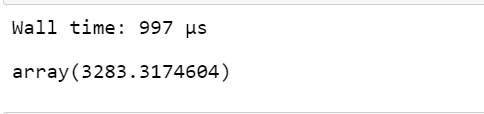## Binned Statistics in Vaex:

Vaex provides a faster alternative to pandas’s groupby as ‘binby’ which can calculate statistics on a regular N-dimensional grid swiftly in regular bins.

## Python3

 `%``time df_vaex.count(binby``=``df_vaex.column7,``                    ``limits``=``[``0``, ``20``], shape``=``10``)`

Output:### Fast Visualization in Vaex:

Visualization of the large dataset is a tedious task. But Vaex can compute these visualizations pretty quickly. The dataset gives a better idea of data distribution when computed in bins and Vaex excels in group aggregate properties, selections, and bins. So, Vaex is able to visualize swiftly and interactively. By Vaex, visualizations can be done even in 3-dimensions on large datasets.
Let’s plot a simple 1-dimensional graph:

## Python3

 `%``time df_vaex.viz.histogram(df_vaex.column1, ``                            ``limits ``=` `[``0``, ``20``])`

Output: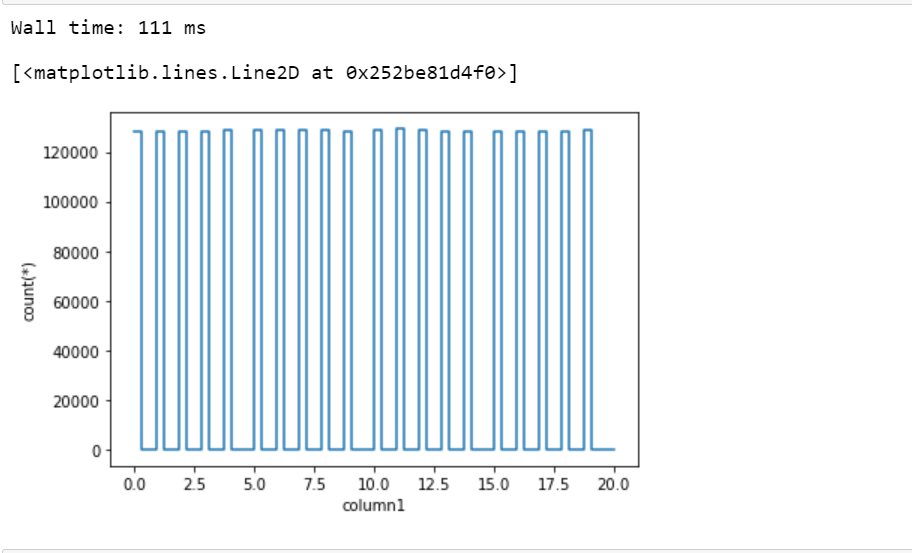## Python

 `df_vaex.viz.heatmap(df_vaex.column7, df_vaex.column8 ``+``                    ``df_vaex.column9, limits``=``[``-``3``, ``20``])`

Output: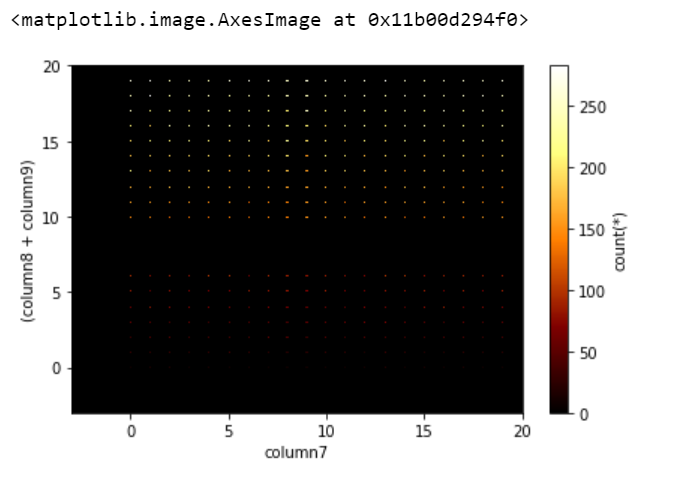We can add statistics expression and visualize by passing the “what=<statistic>(<expression>)” argument. So let’s perform a slightly complicated visualization:

## Python3

 `df_vaex.viz.heatmap(df_vaex.column1, df_vaex.column2,``                    ``what``=``(vaex.stat.mean(df_vaex.column4) ``/``                          ``vaex.stat.std(df_vaex.column4)),``                    ``limits``=``'99.7%'``)`

Output: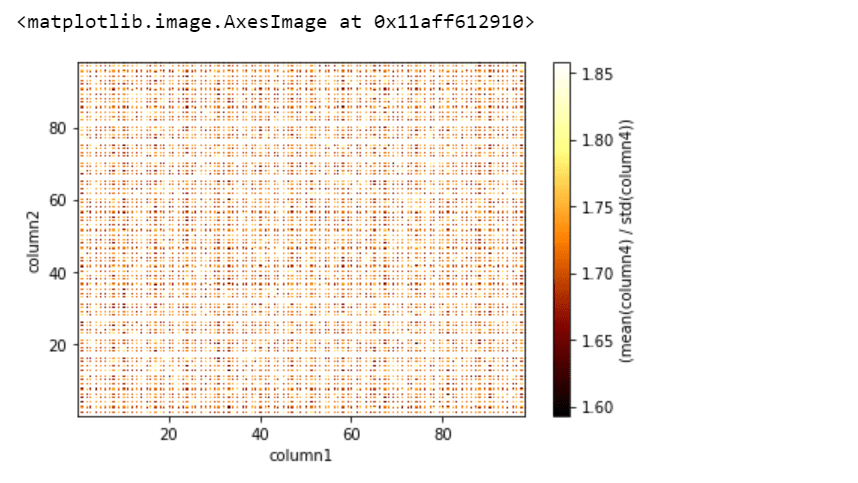Here, the ‘vaex.stat.<statistic>’ objects are very similar to Vaex expressions, which represent an underlying calculation, and also we can apply typical arithmetic and Numpy functions to these calculations.

My Personal Notes arrow_drop_up